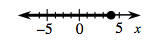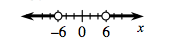### Home > A2C > Chapter 5 > Lesson 5.2.2 > Problem5-80

5-80.

Solve each of the following inequalities. Express the solutions algebraically and on a number line.

1. $3x-5\le7$

Solve the equation $3x-5\le7$ for $x$. Plot the boundary point on a number line. Test a point on each side of the boundary point. Shade the region that makes the inequality true.

$x\le4$1. $x^2+6>42$

See part (a). This inequality is quadratic, so look for two boundary points.

$x<−6$ or $x>6$